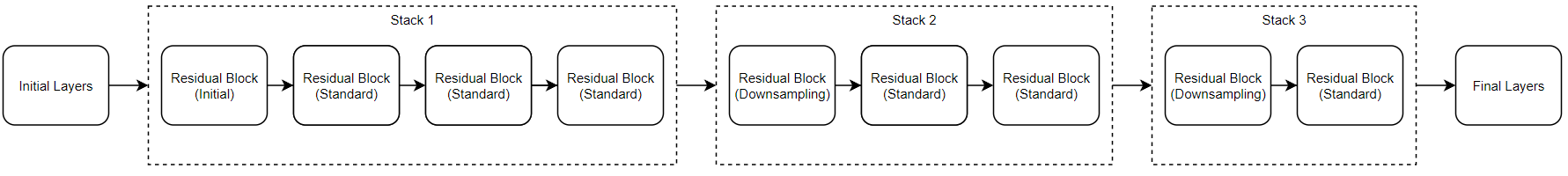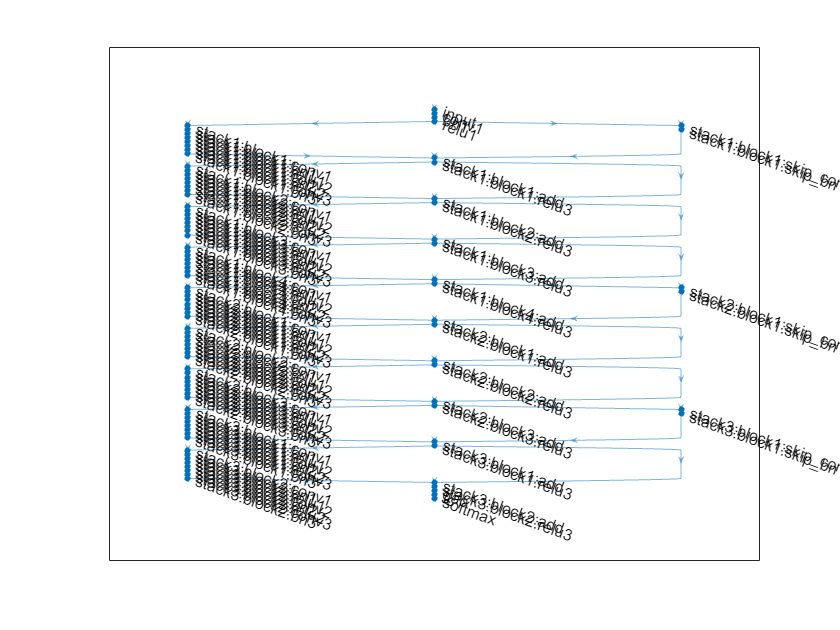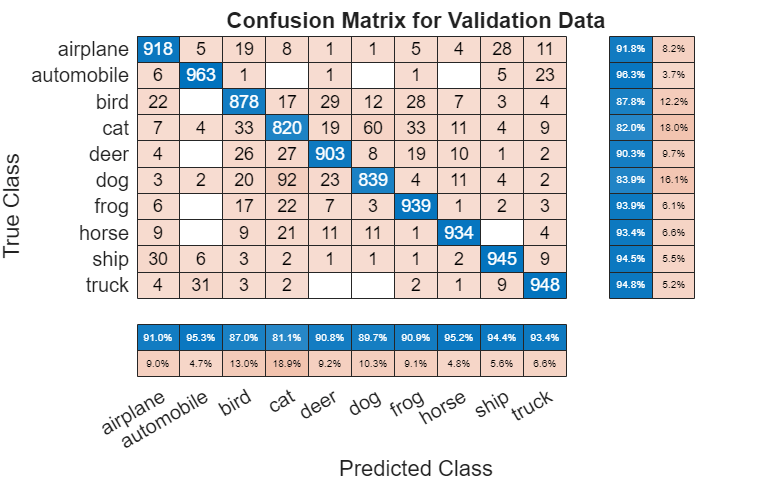# 训练残差网络进行图像分类

ResNet 架构由初始层、后跟包含残差块堆栈以及最终层组成。有三种类型的残差块：

• 初始残差块 - 此块出现在第一个堆栈的开头。此示例使用瓶颈组件；因此，此块包含与下采样块相同的层，但仅在第一个卷积层中具有 `[1,1]` 的步幅。有关详细信息，请参阅 `resnetLayers`

• 标准残差块 - 此块出现在每个堆栈中的第一个下采样残差块后。此块在每个堆栈中出现多次，并保留激活的维度大小。

• 下采样残差块 - 此块出现在每个堆栈的开头（第一个堆栈除外），并且在每个堆栈中只出现一次。下采样块中的第一个卷积单元会将空间维度下采样二分之一。• 使用 `resnetLayers` 函数创建一个残差网络。

• 使用 `trainNetwork` 函数训练网络。经过训练的网络是一个 `DAGNetwork` 对象。

• 使用 `classify``predict` 函数对新数据执行分类和预测。

### 准备数据

```datadir = tempdir; downloadCIFARData(datadir);```

`[XTrain,TTrain,XValidation,TValidation] = loadCIFARData(datadir);`

```figure; idx = randperm(size(XTrain,4),20); im = imtile(XTrain(:,:,:,idx),ThumbnailSize=[96,96]); imshow(im) ```

```imageSize = [32 32 3]; pixelRange = [-4 4]; imageAugmenter = imageDataAugmenter( ... RandXReflection=true, ... RandXTranslation=pixelRange, ... RandYTranslation=pixelRange); augimdsTrain = augmentedImageDatastore(imageSize,XTrain,TTrain, ... DataAugmentation=imageAugmenter, ... OutputSizeMode="randcrop");```

### 定义网络架构

• CIFAR-10 图像是 32×32 像素，因此，使用一个大小为 3 的初始滤波器和大小为 1 的初始步幅。将初始滤波器的数量设置为 16。

• 网络中的第一个堆栈以初始残差块开始。每个后续堆栈从下采样残差块开始。下采样分块中的第一个卷积单元以会将空间维度下采样二分之一。为了使整个网络中每个卷积层所需的计算量大致相同，每次执行空间下采样时，都将滤波器的数量增加一倍。将堆栈深度设置为 `[4 3 2]`，将滤波器数量设置为 `[16 32 64]`

```initialFilterSize = 3; numInitialFilters = 16; initialStride = 1; numFilters = [16 32 64]; stackDepth = [4 3 2]; lgraph = resnetLayers(imageSize,10, ... InitialFilterSize=initialFilterSize, ... InitialNumFilters=numInitialFilters, ... InitialStride=initialStride, ... InitialPoolingLayer="none", ... StackDepth=[4 3 2], ... NumFilters=[16 32 64]);```

`plot(lgraph);`### 训练选项

```miniBatchSize = 128; learnRate = 0.1*miniBatchSize/128; valFrequency = floor(size(XTrain,4)/miniBatchSize); options = trainingOptions("sgdm", ... InitialLearnRate=learnRate, ... MaxEpochs=80, ... MiniBatchSize=miniBatchSize, ... VerboseFrequency=valFrequency, ... Shuffle="every-epoch", ... Plots="training-progress", ... Verbose=false, ... ValidationData={XValidation,TValidation}, ... ValidationFrequency=valFrequency, ... LearnRateSchedule="piecewise", ... LearnRateDropFactor=0.1, ... LearnRateDropPeriod=60);```

### 训练网络

```doTraining = false; if doTraining net = trainNetwork(augimdsTrain,lgraph,options); else load("trainedResidualNetwork.mat","net"); end```### 评估经过训练的网络

```[YValPred,probs] = classify(net,XValidation); validationError = mean(YValPred ~= TValidation); YTrainPred = classify(net,XTrain); trainError = mean(YTrainPred ~= TTrain); disp("Training error: " + trainError*100 + "%")```
```Training error: 3.462% ```
`disp("Validation error: " + validationError*100 + "%")`
```Validation error: 9.27% ```

```figure(Units="normalized",Position=[0.2 0.2 0.4 0.4]); cm = confusionchart(TValidation,YValPred); cm.Title = "Confusion Matrix for Validation Data"; cm.ColumnSummary = "column-normalized"; cm.RowSummary = "row-normalized";``````figure idx = randperm(size(XValidation,4),9); for i = 1:numel(idx) subplot(3,3,i) imshow(XValidation(:,:,:,idx(i))); prob = num2str(100*max(probs(idx(i),:)),3); predClass = char(YValPred(idx(i))); title([predClass + ", " + prob + "%"]) end ```

 Krizhevsky, Alex. "Learning multiple layers of features from tiny images." (2009). https://www.cs.toronto.edu/~kriz/learning-features-2009-TR.pdf

 He, Kaiming, Xiangyu Zhang, Shaoqing Ren, and Jian Sun. "Deep residual learning for image recognition." In Proceedings of the IEEE conference on computer vision and pattern recognition, pp. 770-778. 2016.